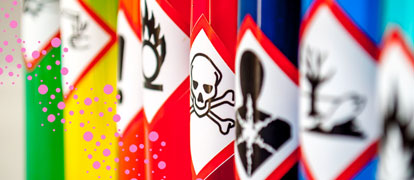# Chemical Reactions Year 11 Chemistry Students Must Know

A helpful list of essential chemical reactions that a Year 11 student should know.## Metals in Water

Reactive Metal + Liquid Water → Metal Hydroxide + Hydrogen Gas

E.g. Na(s) + H2O(l) → NaOH(aq) + H2(g)

Metal + Steam → Metal Oxide + Hydrogen Gas

E.g. Zn(s) + H2O(g) → ZnO(s) + H2(g)

## Combustion of a Metal

Metal + Oxygen Gas → Metal Oxide

E.g. 2Mg(s) + O2(g) → MgO(s)

## Combustion of a Carbon Compound

Hydrocarbon + Oxygen → Carbon Dioxide Gas + Liquid Water

E.g. 2C8H18(l) + 25O2(g) → 16CO2(g) + 18H2O(l)

## Metals with Acids

Metal + Acid → Salt + Hydrogen gas

E.g. Zn(s) + 2HCl(aq) → ZnCl2(aq) + H2(g)

## Carbonates with Acids

Carbonate + Acid → Salt + Carbon Dioxide Gas + Liquid Water

E.g. Na2CO3(aq) + 2HCl(aq) → 2NaCl(aq) + CO2(g) + H2O(l)

## Oxidation

Metal → Metal Ion + Electron/s

E.g. Cu(s) → Cu2+(aq) + 2e

## Reduction

Metal Ion + Electron/s → Metal

E.g. Ag+(aq) + e  Ag(s)

## Redox (Reduction-Oxidation Reactions)

This reaction involves the transfer of electron/s from one reactant to another. This thus results in both a reduction and oxidation reaction occurring. To obtain the redox reaction, you can either write the net ionic equation for a displacement reaction (option 1) or add reduction and oxidation reactions together (option 2).

E.g. OPTION 1:

Cu(s) + 2AgNO3(aq) → Cu(NO3)2(aq) + 2Ag(s)

Cu(s) + 2Ag+(aq) + 2NO3  → Cu2+(aq) + 2NO3 + 2Ag(s)

Cu(s) + 2Ag+(aq) + 2NO3 → Cu2+(aq) + 2NO3 + 2Ag(s)

Cu(s) + 2Ag+(aq) → Cu2+(aq) + 2Ag(s)

E.g. OPTION 2

NOTE: BOTH THE CONSERVATION OF MASS AND CHARGE MUST BE SHOWN IN YOUR EQUATION!

Cu(s) → Cu2+(aq) + 2e

+

2Ag+(aq) + 2e → 2Ag(s)

__________________

Cu(s) + 2Ag+(aq) + 2e → Cu2+(aq) + 2e + 2Ag(s)

Cu(s) + 2Ag+(aq) → Cu2+(aq) + 2Ag(s)

## Photosynthesis

Carbon Dioxide Gas + Liquid Water  Solid Glucose + Oxygen Gas

I.e. 6CO2(g) + 6H2O(l) → C6H12O6(s) + 6O2(g)

## Respiration

Aqueous Glucose + Oxygen Gas  Carbon Dioxide Gas + Liquid Water

I.e. C6H12O6(s) + 6O2(g) → 6CO2(g) + 6H2O(l)

## Lime Water Test (Lime Water and Carbon Dioxide)

Calcium hydroxide + Gaseous carbon dioxide  Solid calcium carbonate + liquid water

I.e. Ca(OH)2(aq) + CO2(g) → CaCO3(s) + H2O(l)

Find out more about the Matrix Year 11 Chemistry Course or Matrix Year 12 Chemistry Course. Matrix courses are available for HSC English, Maths, Physics, Biology and Chemistry.

## Want to Achieve Your ATAR Goal?

• Read All About ATAR & Scaling to learn how to achieve your ATAR goal.
• Join over 4000 students who already have a head start. Book your free lesson now.

### Get free study tips and resources delivered to your inbox.

Join 75,893 students who already have a head start.

Our website uses cookies to provide you with a better browsing experience. If you continue to use this site, you consent to our use of cookies. Read our cookies statement.